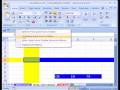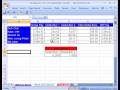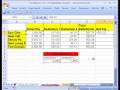HOME

Microsoft Excel TutorialExcel Basics #1: What Is Excel? #1 Video topics:1)What is Excel? Calculating formulas and Data Analysis2)Rows, Columns, Cells, Worksheets, Sheet Tab Names, Workbook3)File extensions (file types): .xlsx, .xlsm, .xls, .xlsb4)Excel 2007 Ribbons5)Excel 2007 Quick Access Tool Bar (QAT)6)How to find features in Excel 20077)How to add buttons to the QAT Quick Access ToolbarExcel Basics #2: Keyboard Shortcuts #2 Video topics:1)Ctrl + C = Copy2)Ctrl + V = Paste3)Ctrl + X = Cut4)Excel 2007 Screen Tips with keyboard shortcuts5)Ctrl + F2 = Print Preview6)How to Discover all the Alt keyboard shortcuts (Excel 2003 and 2007)7)Excel 2003 Alt + F + U = Page Setup8)Excel 2007 Alt P + S + P = Page Setup9)Ctrl + Arrows keys = Move to end of current region10)Ctrl + Home = select cell A111)Ctrl + Roller-ball-on-mouse = Zoom12)Atl + = is the keyboard for Auto SUM13)Shift + Click selects range between to cells14)Ctrl + Shift + arrow = Select whole current region (This trick is the best Excl keyboard shortcut)Excel Basics #3: Formulas w Cell References #3 Video topics:1)Formulas2)Equal sign =3)Ampersand &4)Formulas with Mouse5)Formulas with Arrow Keys6)Formulas with cell references7)Relative Cell References8)Change input numbers in cells and see resulting formulas update instantly (Bricklin and Frankston VisiCalc)9)Copy formulas10)Calculating number result formulas11)Text formulas12)Fill handle (Angry Rabbit) and quick formula copy13)Join cell content and text (using quotes) in text formula using the ampersand &